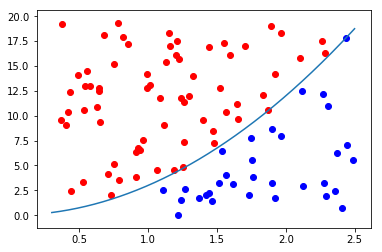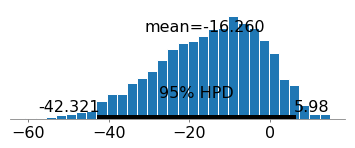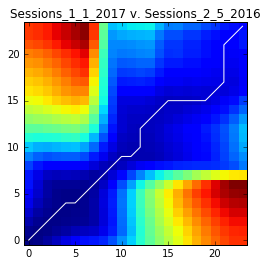### Monte Carlo Integration in PythonMy last post was pretty intense, so I thought that I would drop back down to something a little bit simpler for this post, before I go off and start in on the March Madness stuff again. It turns out […]

### Bayesian Logistic Regression in Python using PYMC3In my last post I talked about bayesian linear regression. A fairly straightforward extension of bayesian linear regression is bayesian logistic regression. Actually, it is incredibly simple to do bayesian logistic regression. If you were following the last post that […]